Mathematical and Physical Journal
for High Schools
Issued by the MATFUND Foundation
 Already signed up? New to KöMaL?

# KöMaL Problems in Physics, May 2005

Show/hide problems of signs:## Problems with sign 'M'

Deadline expired on June 13, 2005.

M. 261. Create a hair hygrometer to measure the relative humidity of air. Take measurements in various rooms of your flat and also in the open air at different times of the day.

(6 pont)

statistics## Problems with sign 'P'

Deadline expired on June 13, 2005.

P. 3803. The heater filaments of an electric cooker are connected as is shown in the figure. If we connect voltage between points A and B we can boil 500 g of water in a certain time. How much water can be boiled during the same time if we put the voltage between points A and C? (Let us assume that the heater filaments only heat the water and the initial temperature of the water is the same in both cases.)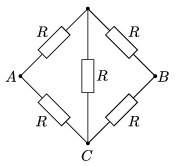(3 pont)

statistics

P. 3804. The propellers of aircrafts are usually positioned at the front to pull the craft. On the other hand the propellers of ships are always mounted on the aft, so they push ships forward. What can be the explanation of the different implementations?

(4 pont)

statistics

P. 3805. A small ball hanging on a thread revolves in a vertical plane. At the uppermost point of its path its velocity is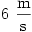. The force stretching the thread is three times as much in the lowermost point than in the uppermost one.

a) How long is the thread?

b) What is the highest velocity of the ball?

(4 pont)

statistics

P. 3806. A soldier has to cross a 60 m wide river on a ropeway grabbing on a pulley. The length of the rope is 75 m, its ends are fixed at the same height on both banks of the river. The soldier, whose mass together with his combat gear is 90 kg, starts from a platform under one of the fixed points with zero initial velocity.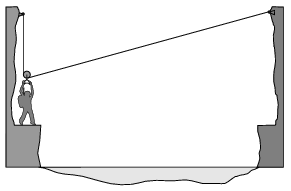a) What is the highest velocity of the soldier during the crossing?

b) What is the force exerted on the rope at that moment? (Take the rope as inextensible, weightless and free of any friction.)

(5 pont)

statistics

P. 3807. We press a straight circular cork cone of height h to the bottom of a glass vessel.

Then we carefully pour mercury onto it. The density of cork is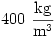. We pour the mercury until the pressing force exerted on the bottom of the vessel of the cork cone already let loose is twice that of its weight. What is the height of the mercury at this time in the vessel?

(5 pont)

statistics

P. 3808. In a perfectly heat-insulated tank of solid walls there is nitrogen gas of a temperature of 300 K. The tank initially moves with a velocity of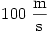and then stops

a) suddenly.

b) in a certain time decelerating uniformly.

Does the temperature of the gas change, and if it does, how?

(5 pont)

statistics

P. 3809. Two identical solenoids are closely packed with a common axle. The cross section of the coils is A and there are n turns of the coil in the unit length. What is the force between them if we have

a) current I in both of them?

b) current I1 in one and I2 in the other?

(5 pont)

statistics

P. 3810. There is electric current I flowing in a very long circular pipe conductor. What is the magnitude of the magnetic induction vector inside the hollow?

(4 pont)

statistics

P. 3811. A charged particle travels into a region in which there is a friction force proportional to the particle's velocity. It travels 10 cm from the point of entry into the region before coming to rest. If a magnetic field of unknown strength is turned on in the region, the particle comes to rest at 6 cm from the point of entry. How far from the point of entry will the particle come to rest if the magnetic field is doubled?

(5 pont)

statistics

P. 3812. A positron collides into an electron of negligible velocity. The velocities of the two photons created in the pair annihilation are perpendicular to each other. What was the velocity of the positron at the least?

(4 pont)

statistics